# NCERT Solutions for Class 10 Maths Exercise 7.1

Get Free NCERT Solutions for Class 10 Maths Chapter 7 Ex 7.1 Coordinate Geometry Class 10 Maths NCERT Solutions are extremely helpful while doing homework. Exercise 7.1 Class 10 Maths NCERT Solutions were prepared by Experienced LearnCBSE.in Teachers. Detailed answers of all the questions in Chapter 7 Maths Class 10 Coordinate Geometry Exercise 7.1 Provided in NCERT Textbook

NCERT Solutions For Class 10 Maths Chapter 7 Coordinate Geometry Ex 7.1
NCERT Solutions for Class 10 Maths Chapter 7 Coordinate Geometry Ex 7.1 are part of NCERT Solutions for Class 10 Maths. Here we have given NCERT Solutions for Class 10 Maths Chapter 7 Coordinate Geometry Ex 7.1.

## NCERT Solutions for Class 10 Maths Exercise 7.1

Exercise 7.1 Class 10 Question 1
Find the distance between the following pairs of points:
(i) (2,3), (4,1)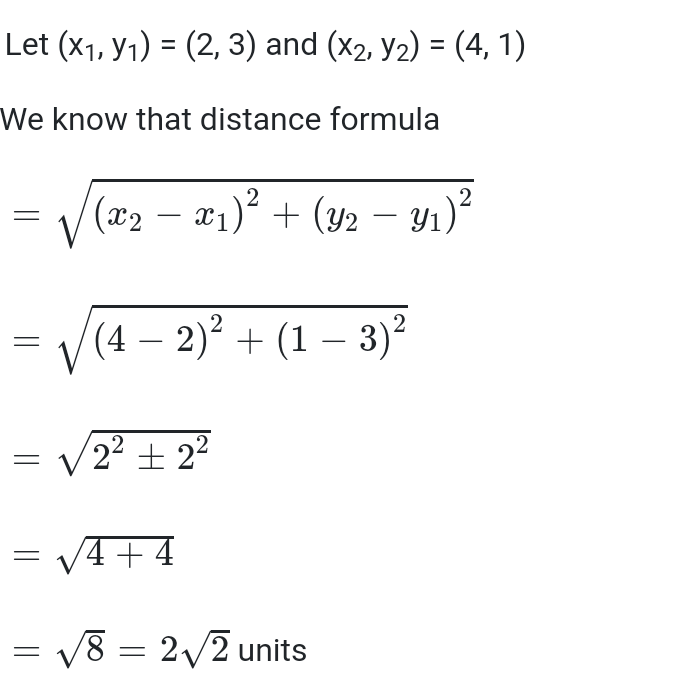(ii) (-5, 7), (-1, 3)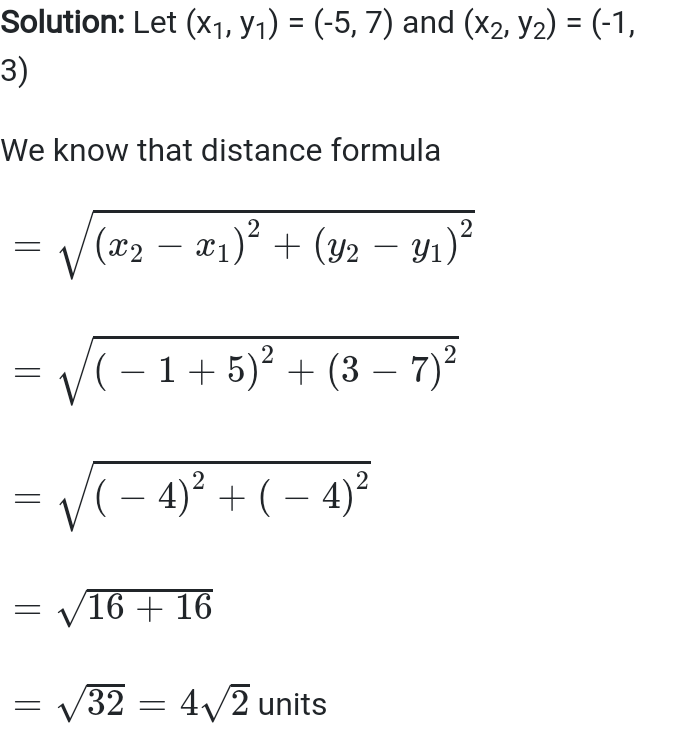(iii) (a, b), (-a, -b)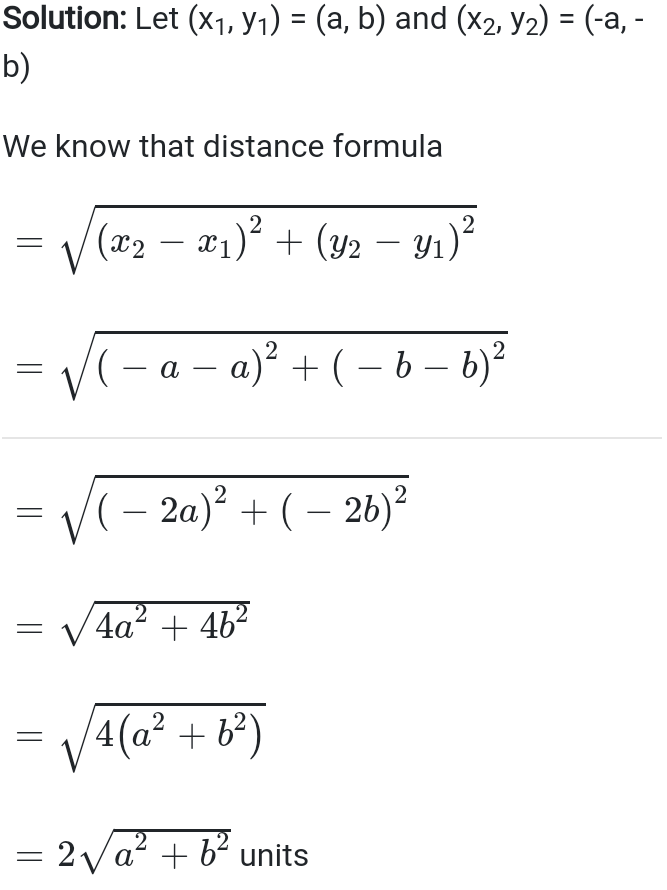Exercise 7.1 Class 10 Question 2
Find the distance between the points (0, 0) and (36, 15). Can you now find the distance between the two towns A and B discussed in section 7.2 and shown in following figure.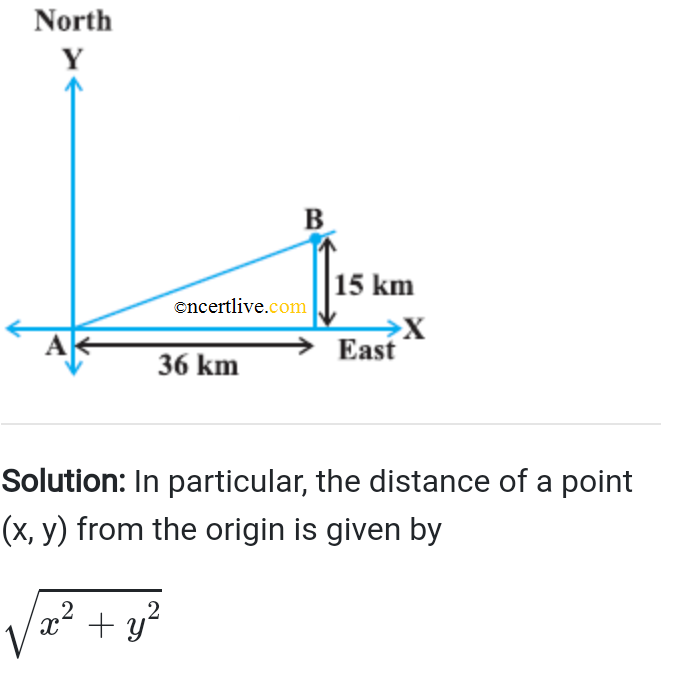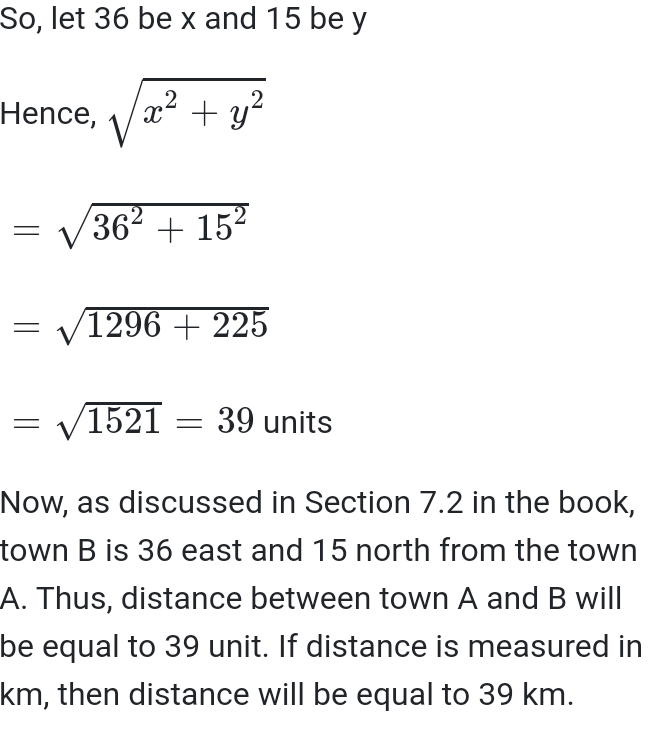Exercise 7.1 Class 10 Question 3
Determine if the points (1, 5), (2, 3) and (–2, –11) are collinear.

Exercise 7.1 Class 10 Question 4
Check whether (5, 2), (6, 4) and (7, – 2) are the vertices of an isosceles triangle.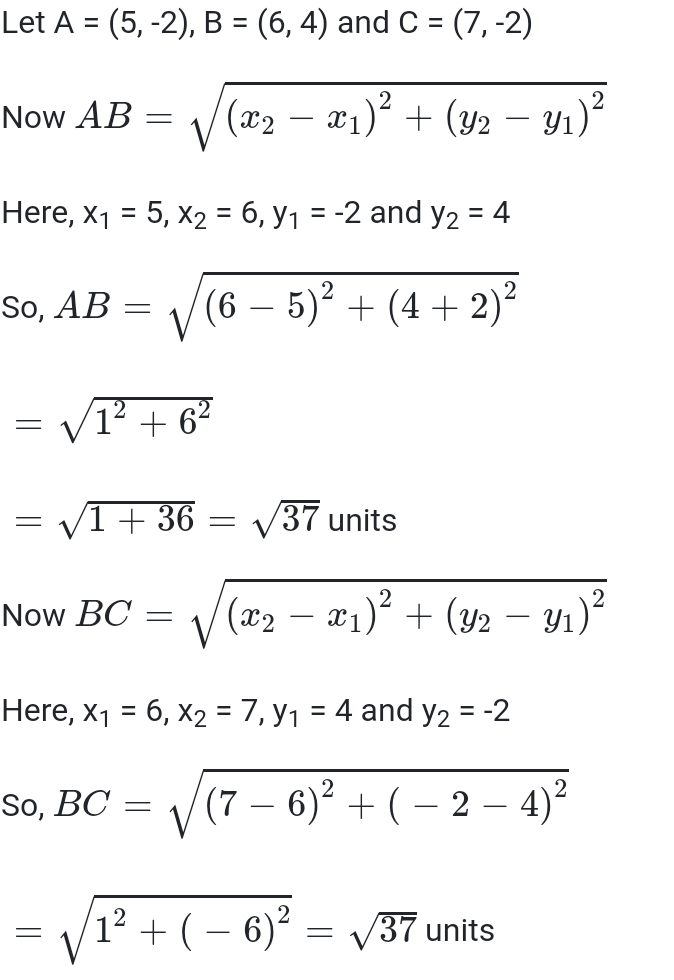Exercise 7.1 Class 10 Question 5
In a classroom, 4 friends are seated at the points A, B, C and D as shown in Figure 7.8. Champa and Chameli walk into the class and after observing for a few minutes Champa as Chameli, “Don’t you think ABCD is square?” Chameli disagrees. Using distance formula find which of them is correct.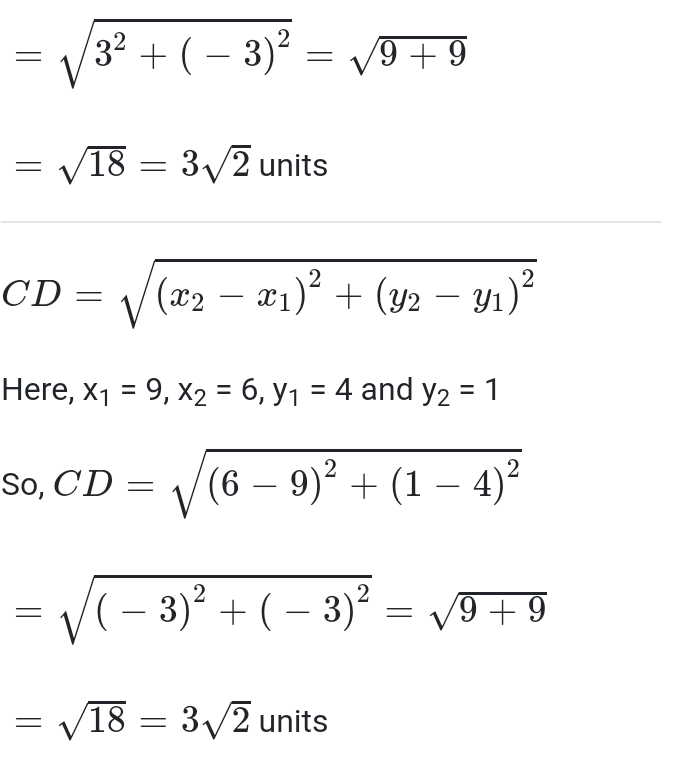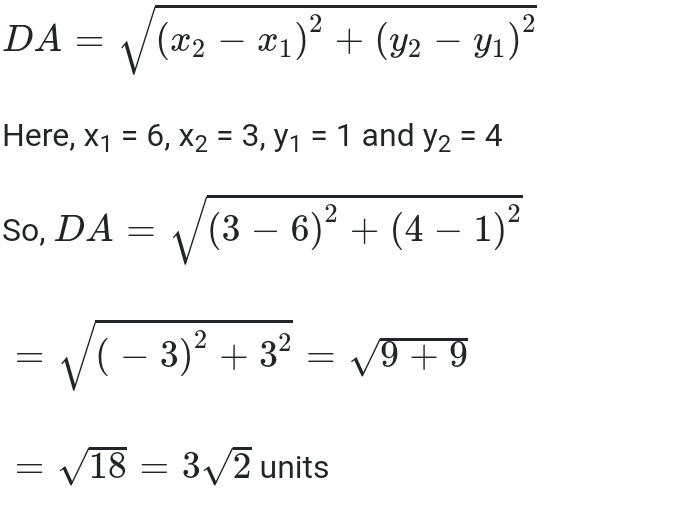Exercise 7.1 Class 10 Question 6
Name the type of quadrilateral formed, if any, by the following points, and give reasons for your answer.
(i) (-1, -2), (1, 0), (-1, 2), (-3,0)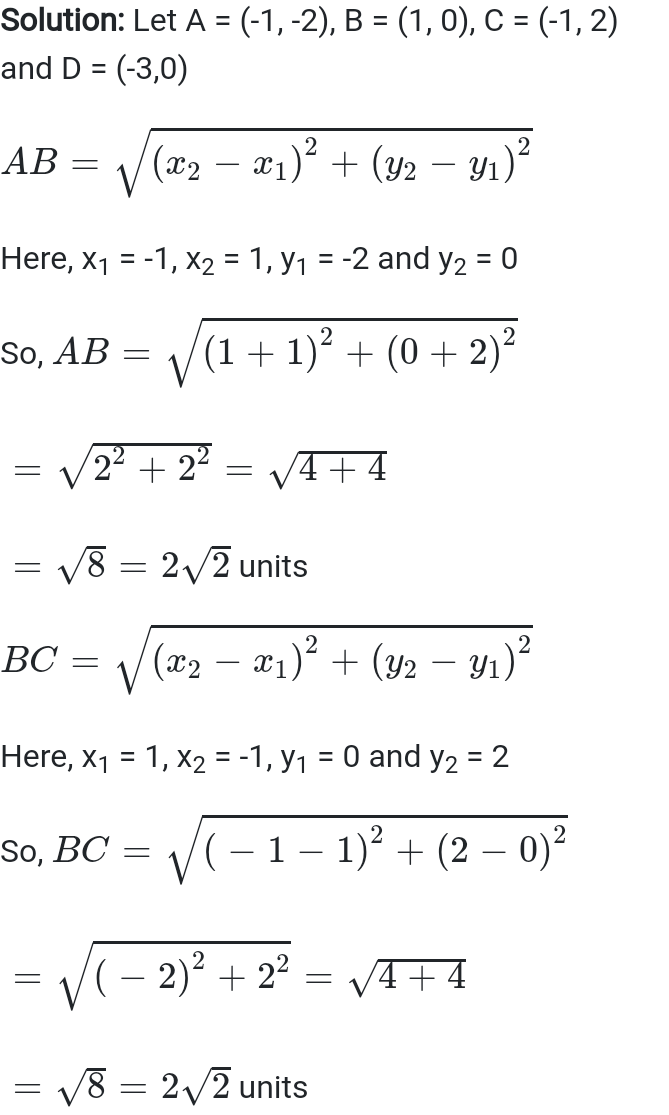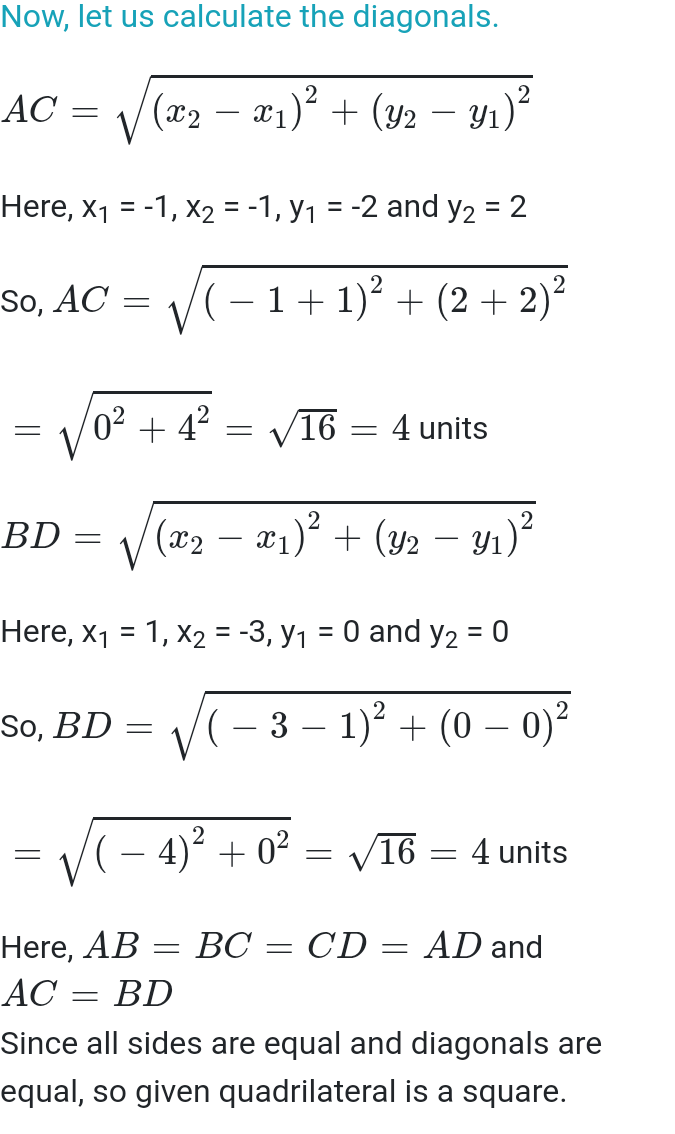(ii) (-3, 5), (3, 1), (0, 3), (-1,-4)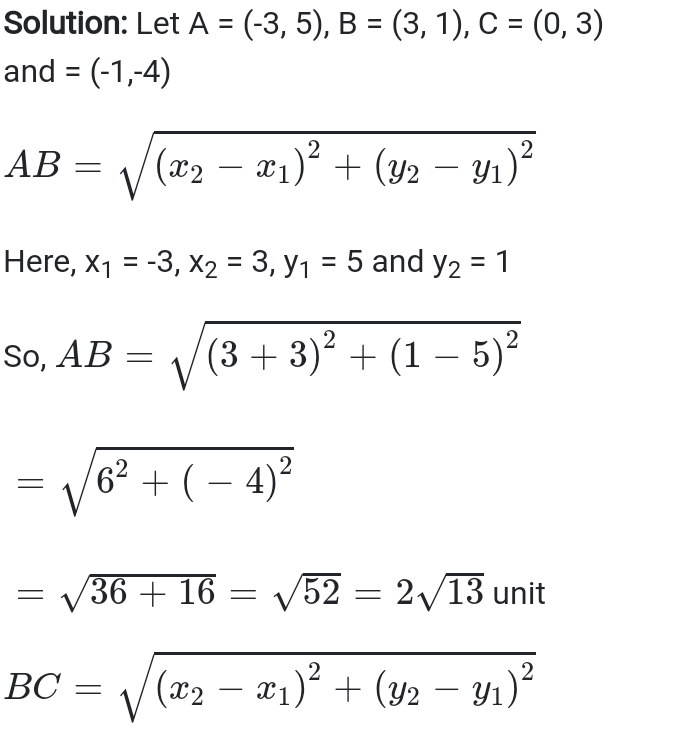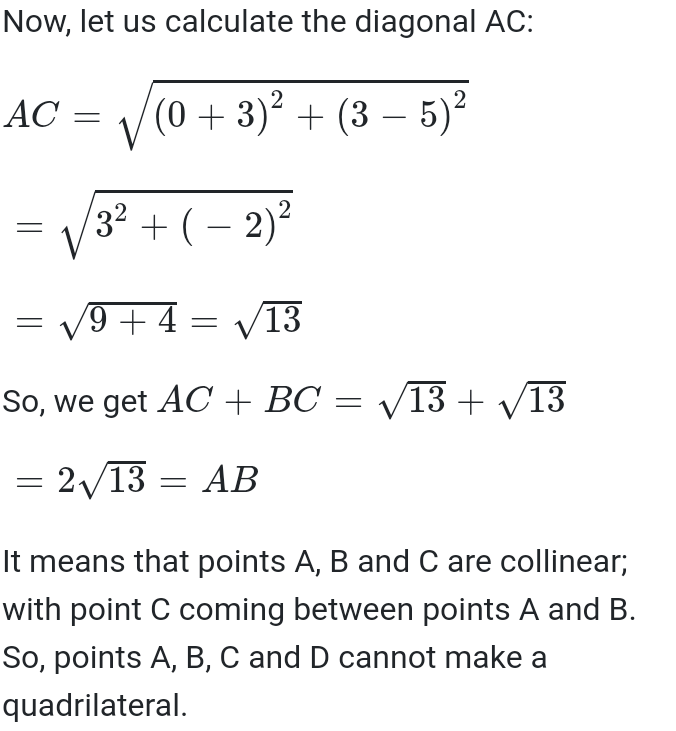(iii) (4, 5), (7, 6), (4, 3), (1, 2)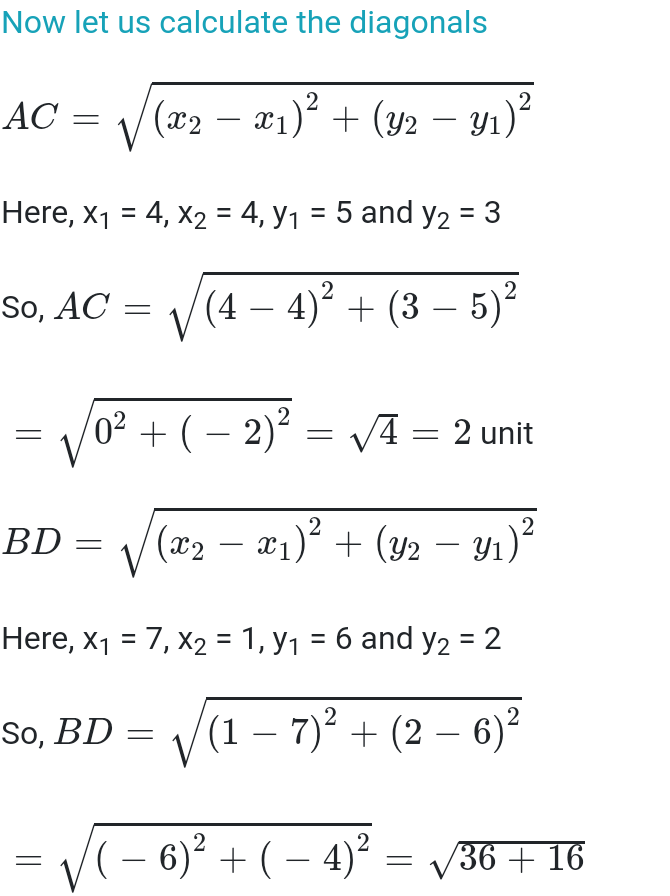Exercise 7.1 Class 10 Question 7
Find the point on the x-axis which is equidistant from (2, –5) and (– 2, 9).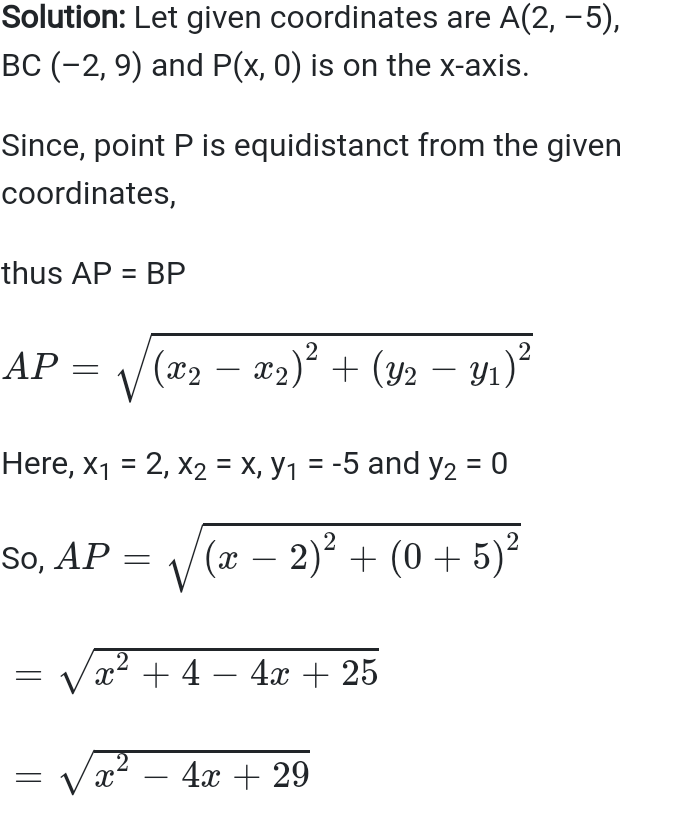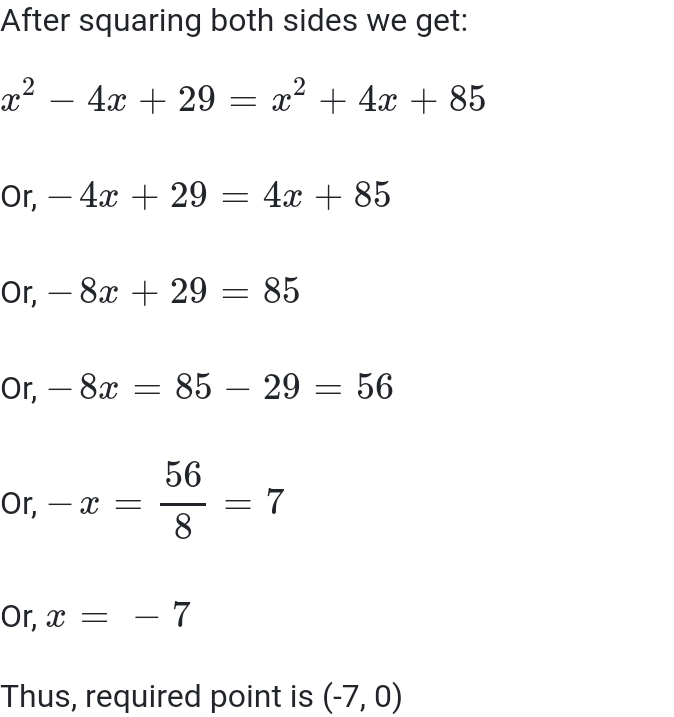Exercise 7.1 Class 10 Question 8
Find the value of y for the distance between the points P(2, – 3) and Q (10, y) is 10 units.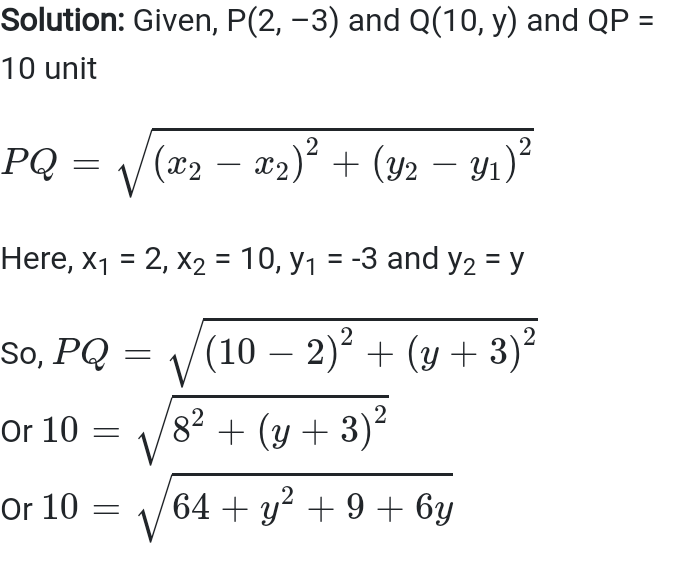Exercise 7.1 Class 10 Question 9
If Q (0, 1) is equidistant from P(5, – 3) and R (x, 6), find the value of x. Also find the distances QR and PR.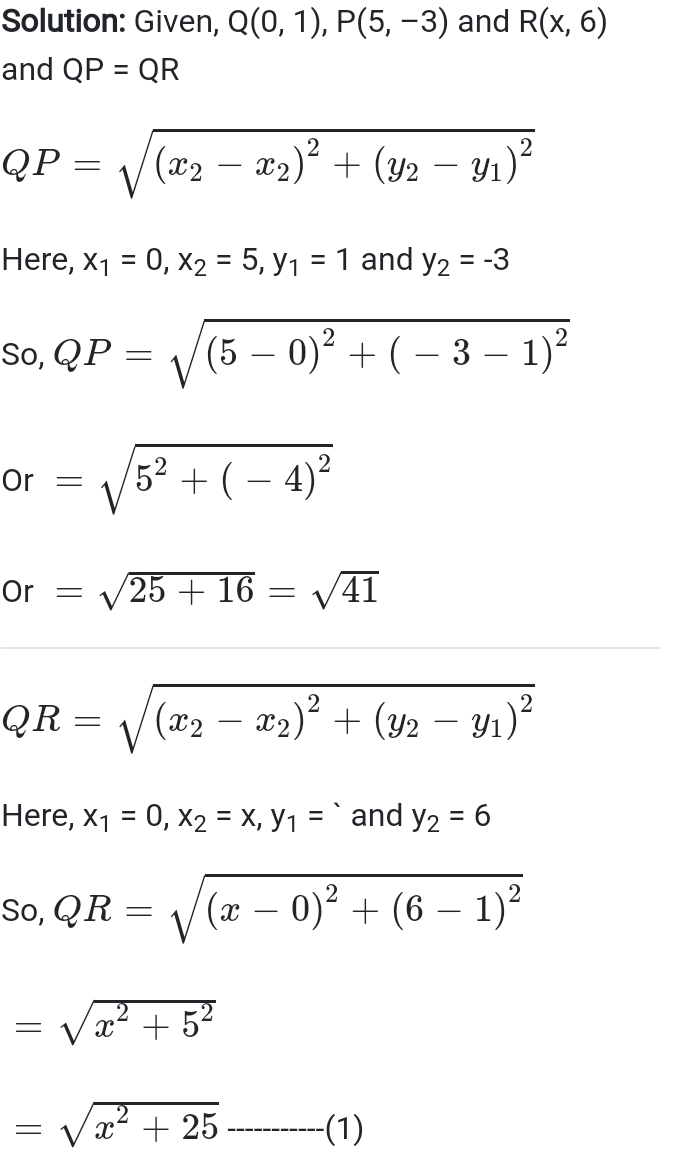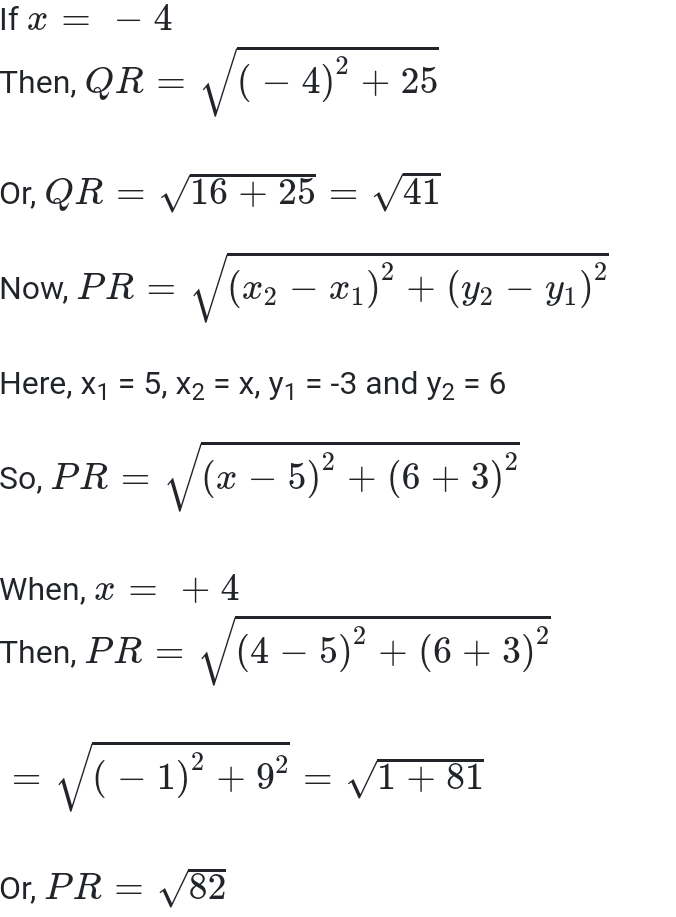Exercise 7.1 Class 10 Question 10
Find the relation between x and y such that the point (x, y) is equidistance from the point (3, 6) and (–3, 4).
Access other exercise solutions of class 10 Maths Chapter 7- Coordinate Geometry
Exercise 7.2– 10 Questions ( 8 long answer questions, 2 short answer questions)
Exercise 7.3– 5 Questions (3 long answer questions, 2 practical based questions)
Exercise 7.4– 8 Questions (6 long answer questions, 1 practical based question, 1 reasoning question)

The exercise solutions provided here are created with a vision to assist the students in their first term exam preparation. Exercise solution cover all the questions given in Exercise 7.1 of the NCERT textbook. These solutions are researched and prepared by our subject experts. Studying this study material will aid you in solving different questions on distance from a point.

Exercise solutions are provided in PDF format for your easy access and download. By studying this solution you can clear your doubts on distance calculation. By studying this study material you can get well acquainted with all important formulas. Here, you can also get knowledge of alternative methods to solve distance from a point problems.

Topics covered in Exercise 7.1
• Introduction
• Distance formula